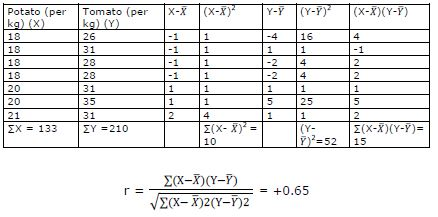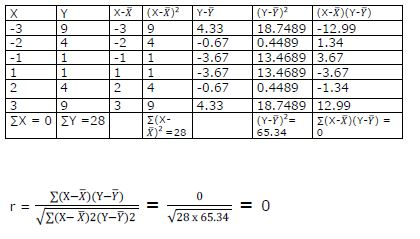# NCERT Solution Class 11 Statistics Correlation

NCERT Solution for Class 11 Statistics for chapter 7 Correlation

Q1. The unit of correlation coefficient between height in feet and weight in kgs is

(i). Kg/feet

(ii). Percentage

(iii). non-existent

(iii) Non-existent

Explanation: Correlation coefficient has no unit. It is pure numeric term used to measure the degree of association between variables.

Q2. The range of simple correlation coefficient is

(i). 0 to infinity

(ii). Minus one to plus one

(iii). Minus infinity to infinity

(ii) Minus one to plus one

Explanation: The value of correlation coefficient lies between minus one to plus one, if the value lies outside this range it indicates error in calculation.

Q3. If rxy is positive the relation between X and Y is of the type

(i). When Y increases X increases

(ii). When Y decreases X increases

(iii). When Y increases X does not change

(i) When Y increases X increases

Explanation: A positive correlation implies that both the variables move in a similar direction. If there is a increase in X, Y also increases in same direction.

Q4. If rxy = 0 the variable X and Y are

(i). Linearly related

(ii). Not linearly related

(iii). Independent

(ii) Not linearly related

Explanation: If rxy = 0, it means that there is a absence of linear relation between the variables, but there may exist a non linear relation between variables.

Q5. Of the following three measures which can measure any type of relationship

(i). Karl Pearson’s coefficient of correlation

(ii). Spearman’s rank correlation

(iii). Scatter diagram

(ii) Spearman’s rank correlation

Explanation: Spearman’s rank correlation is the measure which can measure any type of relationship. But, Karl Pearson’s coefficient is the most widely used method due to the preciseness of the ‘r’ value.

Q6. If precisely measured data are available the simple correlation coefficient is

(i). More accurate than rank correlation coefficient
(ii). Less accurate than rank correlation coefficient
(iii). as accurate as the rank correlation coefficient

(iii) As accurate as the rank correlation coefficient

Q7. Why is r preferred to covariance as a measure of association?

“r” i.e. correlation coefficient is preferred to covariance as a measure of association because

• r is independent of change in scale and origin.
• r has a specific range (-1 to +1 ), so it comes handy to interpret the results quickly.
• Covariance is a part of correlation coefficient.

Q8. Can r lie outside the -1 and 1 range depending on the type of data?

No, value of r cannot lie outside the range -1 and 1 depending on the type of data. If r = 1 or -1 that means there is a perfect positive or perfect negative relation between variables and if r = 0 that means there isn’t any correlation. But, if value of r lies outside the range -1 and 1, then it indicates that there is an error in calculation.

Q9. Does correlation imply causation?

No, correlation does not imply causation. Correlation only implies association between two variables. It doesn’t represent any cause and effect relation between the two variables. Correlation between two variables only means that two variables are either positively or negatively or neither related at all. It only measures the degree and intensity of the relation between the variables. For example marks scored by a student in exams is correlated to number of days the student went to school but the marks scored by him doesn’t depend on the number of days he went to school.

Q10. When is rank correlation more precise than simple correlation coefficient?

Rank correlation is more precise than simple correlation coefficient in situations when it is required quantify qualities. Ranking is a better alternative for quantification of qualities, which can’t be done in simple correlation. It is also useful when correlation coefficient between two variables with extreme values is quite different from the coefficient without the extreme values. For example, in a beauty contest judges may have to prepare a list of participants in order of their beauty. There is no procedure or numerical system which judges beauty, so in order to prepare a list judges rank the participants based on their features. In this manner rank correlation can be used which gives more precise value than the simple correlation.

Q11. Does zero correlation mean independence?

No, zero correlation doesn’t mean independence. Zero correlation only indicates absence of a linear relation between the variables. There might exist a non-linear relation between them, thus zero correlation necessarily doesn’t mean independence.

Q12. Can simple correlation coefficient measure any type of relationship?

No, simple correlation is not able to measure all the types of relationship. It can only measure linear relationships between the variables. It is not able to interpret the non-linear relationships between the variables. In case correlation coefficient returns the value zero it either means there is no correlation or there is non- linear relationship which cannot be measured.

Q13. Collect the price of five vegetables from your local market every day for a week. Calculate their correlation coefficients. Interpret the result.

 Day Potato(per kg) Tomato(per kg) Onion (per kg) Babycorn(per kg) Broccoli (per kg) 1 18 30 35 110 170 2 18 35 36 112 165 3 18 32 35 120 150 4 20 32 34 118 155 5 20 35 36 115 155 6 20 35 35 110 160 7 21 32 35 111 165

Correlation between prices of potato and tomatoLikewise we can calculate correlation between different pairs of vegetables or we can use multivariate correlation to find the relation between the vegetables.

Q14. Measure the height of your classmates. Ask them the height of their bench mate. Calculate the correlation coefficient of these two variables. Interpret the result.

(Hypothetical example, results may vary)Correlation coefficient between the height of classmate and height of his bench mate are negatively related.

Q15. List some variables where accurate measurement is difficult.

Some of the variables for which accurate measurement is difficult are:

• Beauty
• Honesty
• Intelligence
• Ability
• Bravery
• Fairness, etc

Q16. Interpret the values of r as 1,-1 and 0

r = 1 implies there is a perfect positive correlation between the variables.

r = -1 implies there is a perfect negative correlation between the variables

r = 0 implies there is no correlation between the variables.

Q17. Why does rank correlation coefficient differ from Pearsonian correlation coefficient?

Rank coefficient differs from pearsonian correlation coefficient because in rank coefficient, specific ranks are assigned to the data which leads to loss of information and all the information regarding the data is not utilised. Only if the data in the rank coefficient method is ranked precisely, it leads to similar values as the pearsonian coefficient. . The value of rank coefficient also differs due to the first differences of the value of items in the series arranged are almost never constant. Generally, both the methods result in same value of ‘r’; but pearsonian coefficient is more widely used than rank coefficient as it utilizes information from the whole series of frequency distribution.

Q18. Calculate the correlation coefficient between the height of fathers in inches (X) and their sons (Y)

 X 65 66 57 67 68 69 70 72 Y 67 56 65 68 72 72 69 71There is a positive correlation between height of fathers and their sons.

Q19. Calculate the correlation coefficient between X and Y and comment on their relationship:

 X -3 -2 -1 1 2 3 Y 9 4 1 1 4 9Correlation coefficient between X and Y is zero. This means that X and Y have no linear relation between them.

(They a have a non-linear relationship Y = X2)

Q20. Calculate the correlation coefficient between X and Y and comment on their relationship

 X 1 3 4 5 7 8 Y 2 6 8 10 14 16Correlation coefficient between X and Y is +1. There is a perfect positive correlation between X and Y. If there is a change in X, then there is equi-proportional change in Y.

## Tags:

Click for more Economics Study Material

## Latest NCERT & CBSE News

Read the latest news and announcements from NCERT and CBSE below. Important updates relating to your studies which will help you to keep yourself updated with latest happenings in school level education. Keep yourself updated with all latest news and also read articles from teachers which will help you to improve your studies, increase motivation level and promote faster learning

### Time management for CBSE students

The first thing to learn about Time Management is that time is theoretical so you can’t really manage it. What you do when you get into time management, is that you manage yourself. You decide what has to be done, when it must be done and how to do it in the stipulated...

### ICSE Board Exams Cancelled

The ICSE (Class X) 2021 Examination: Given the present worsening situation of the Covid- 19 Pandemic in the country, the CISCE has decided to CANCEL the ICSE (Class X) 2021 Examination. The options given in the earlier Circular dated 16th April 2021, now stands...

### CBSE Assessment Framework

The Central Board of Secondary Education (CBSE) today, announced a suggested competency-based assessment framework to strengthen India’s existing school education system for secondary level (classes 6-10) and improve the overall learning outcomes of students across...